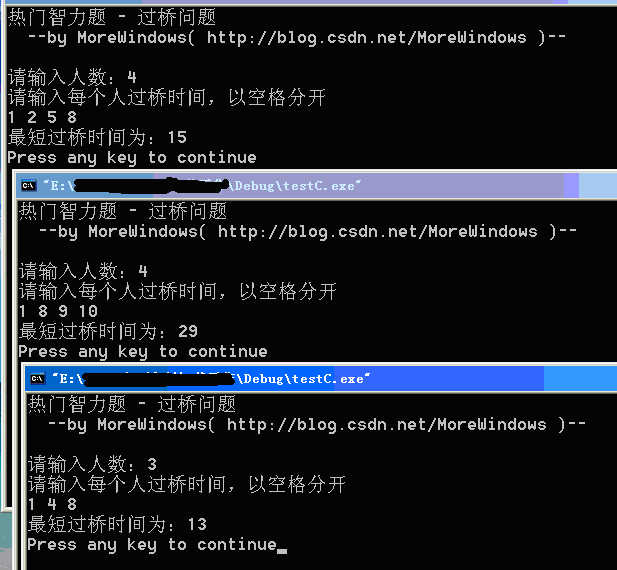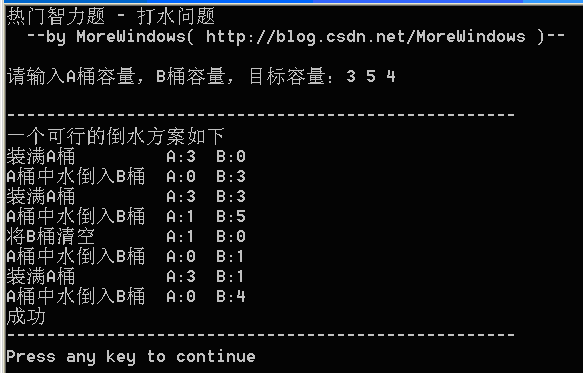# 热门智力题 过桥问题和倒水问题

## 一．过桥问题

```//热门智力题 - 过桥问题
#include<iostream.h>
#include<stdlib.h>
const int MAXN = 1000;
int cmp(const void *x,const void *y)
{
return *(int *)x-*(int *)y;
}
int main()
{
cout<<"热门智力题 - 过桥问题"<<endl;
cout<<"  --by MoreWindows( http://blog.csdn.net/MoreWindows )--\n"<<endl;

int n, i, sum, a[MAXN];
cout<<"请输入人数：";
cin>>n;
cout<<"请输入每个人过桥时间，以空格分开"<<endl;
for (i = 0; i < n; i++)
cin>>a[i];
qsort(a, n, sizeof(a), cmp);
sum = 0;
for (i = n - 1; i > 2; i = i - 2)
{
//最小者将最大2个送走或最小2个将最大2个送走
if (a + a + a + a[i] < a + a + a[i - 1] + a[i])
sum = sum + a + a + a + a[i];
else
sum = sum + a + a + a[i - 1] + a[i];
}
if (i == 2)
sum = sum + a + a + a;
else if (i == 1)
sum = sum + a;
else
sum = a;
cout<<"最短过桥时间为："<<sum<<endl;
return 0;
}```## 二．倒水问题

3 % 5 = 3

6 % 5 = 1

9 % 5 = 4

7 % 11 = 7

14 % 11 = 3

21 % 11 = 10

28 % 11 = 6

35 % 11 = 2

```//热门智力题 - 打水问题
//基本思想：用小桶容量的倍数对大桶的容量进行取余。
//指导方针：不断用小桶装水倒入大桶，大桶满了立即清空，
//每次判断下二个桶中水的容量是否等于指定容量。
#include<iostream>
#include <vector>
#include<string>
using namespace std;
const string OPERATOR_NAME = {
"装满A桶","装满B桶",
"将A桶清空","将B桶清空",
"A桶中水倒入B桶","B桶中水倒入A桶",
"成功"
};
int main()
{
cout<<"热门智力题 - 打水问题"<<endl;
cout<<"  --by MoreWindows( http://blog.csdn.net/MoreWindows )--\n"<<endl;

int    a_volume, b_volume, goal_volume;
vector<string> record;       //记录操作步数
int    ai;
int    i, a_water, b_water;

cout<<"请输入A桶容量，B桶容量，目标容量：";
cin>>a_volume>>b_volume>>goal_volume;
a_water = b_water = 0; //A桶，B桶中有多少升水
char szTemp;
while (true)
{
if (a_water == 0)//A桶没水,就装满水
{
a_water = a_volume;
sprintf(szTemp, "         A:%d  B:%d", a_water, b_water);
record.push_back(OPERATOR_NAME + szTemp);//fill A
}
else
{
//如果A桶的水比(B桶容量-B桶的水)要多
if (a_water > b_volume - b_water)
{
//A桶的水==A桶的水+B桶的水-B桶容量
a_water = a_water + b_water- b_volume;
b_water = b_volume;		 //B桶的水装满了
sprintf(szTemp, "  A:%d  B:%d", a_water, b_water);
record.push_back(OPERATOR_NAME + szTemp);//A->B
if (a_water == goal_volume)
break;
b_water = 0;			//将B桶清空
sprintf(szTemp, "       A:%d  B:%d", a_water, b_water);
record.push_back(OPERATOR_NAME + szTemp);
}
else
{
//B桶的水==A桶的水+B桶的水
b_water += a_water;
a_water = 0;
sprintf(szTemp, "  A:%d  B:%d", a_water, b_water);
record.push_back(OPERATOR_NAME + szTemp);//A->B
if (b_water == goal_volume)
break;
}
}
}
record.push_back(OPERATOR_NAME);	//success
cout<<"\n---------------------------------------------------"<<endl;
cout<<"一个可行的倒水方案如下"<<endl;
vector<string>::iterator pos;
for (pos = record.begin(); pos != record.end(); pos++)
cout<<*pos<<endl;
cout<<"---------------------------------------------------"<<endl;
return 0;
}```